# Breather Surface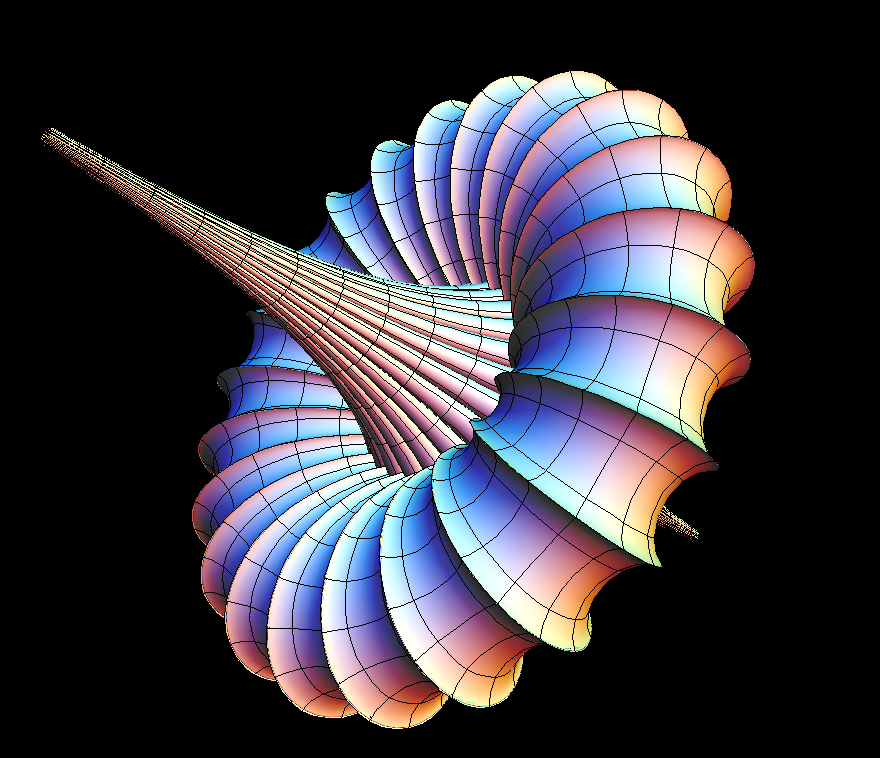Breather Surface family, with changing parameters.

The Breather surface is the surface of Gaussian curvature -1 that corresponds to the following solution of the Sine-Gordon equation:

`q(u,v) := 4 * arctan( (aa / w) * (sin(w * v)) / (cosh(aa * u)) )`

where:

`w := sqrt( 1 - aa * aa)`

Breather Surface can be parametrized by:

```   wsqr := 1 - aa * aa;
w := sqrt(wsqr);
denom := aa * (sqr(w * cosh(aa * u)) + sqr(aa * sin(w * v)))

x = -u + (2 * wsqr * cosh(aa * u) * sinh(aa * u) / denom)
y = 2 * w * cosh(aa * u) * (-(w * cos(v) * cos(w * v)) - (sin(v) * sin(w * v))) / denom
z = 2 * w * cosh(aa * u) * (-(w * sin(v) * cos(w * v)) + (cos(v) * sin(w * v))) / denom
```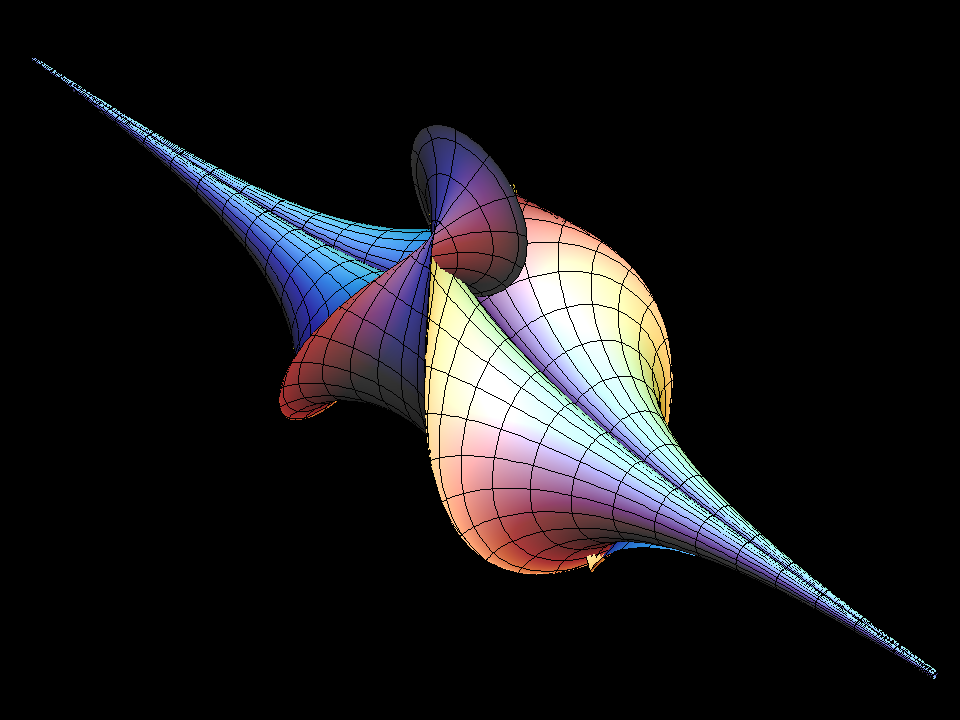The breather family has at one end of its parameter range the Kuen surface as a limit. Our animation starts from the Kuen Surface.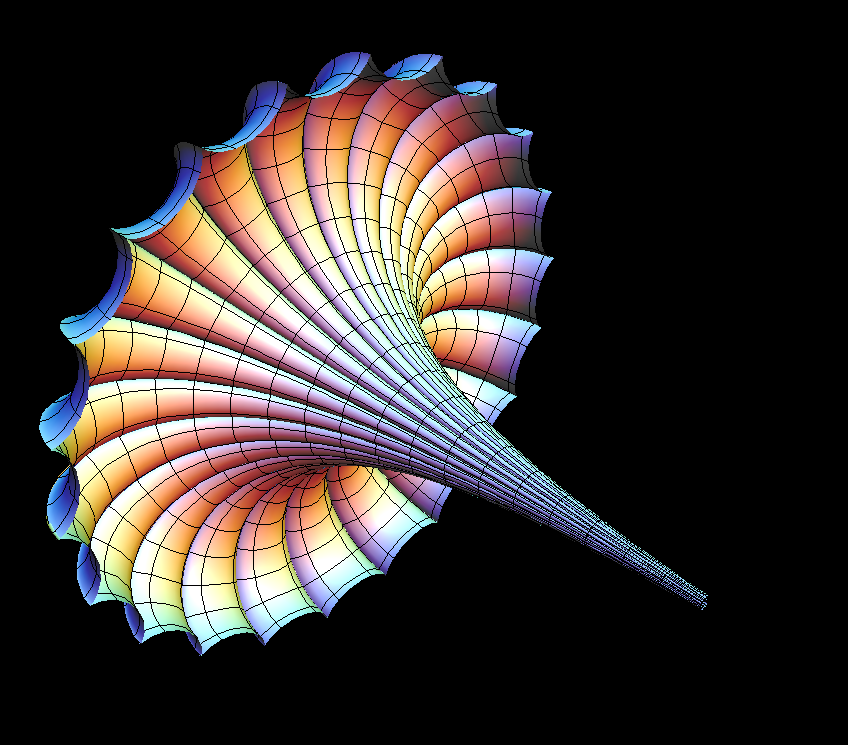Half of Breather, with changing parameters. Certain parameter creates Breather with dihedral symmetry of order 2*k. (k = 22, 8, 3 in the animation)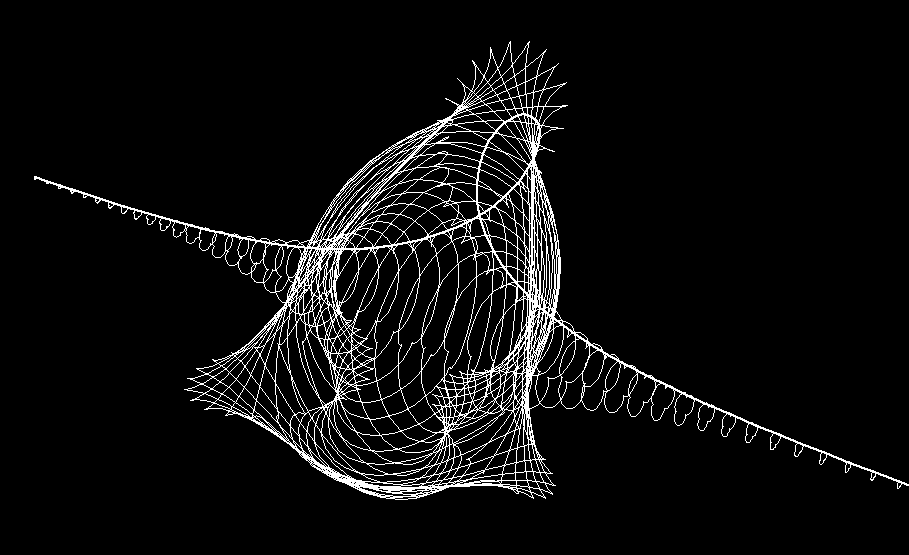Breather Curvature lines. This picture shows how a sine-Gordon soliton solution leads to a construction of the surface: The soliton solution determines ODEs for the curvature lines. This is first used to compute one of the planar curvature lines. Starting from it, a similar ODE determines orthogonal curvature lines.

(Note: curvature lines, are curves on the surface that are formed by the 2 directions of principle curvatures, at each point.)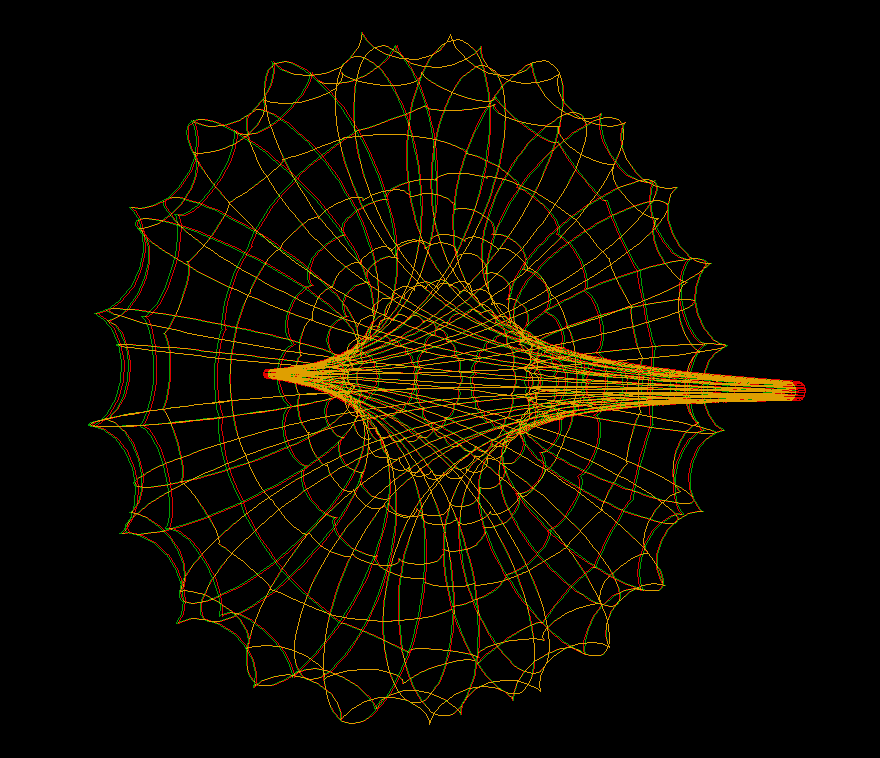One family of curvature lines are planar curves (i.e. each lies on a plane), the planes that pass through the Breather's axis. Dini family of surfaces also has this property. [see Dini Surface]